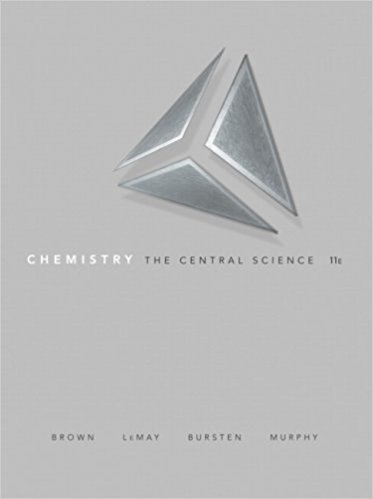×
×

# onsider the A2X4 molecule depicted below, where A and X are elements. The A -A bondISBN: 9780136006176 365

## Solution for problem 7.3 Chapter 7

Chemistry: The Central Science | 11th Edition

• Textbook Solutions
• 2901 Step-by-step solutions solved by professors and subject experts
• Get 24/7 help from StudySoup virtual teaching assistantsChemistry: The Central Science | 11th Edition

4 5 1 414 Reviews
18
4
Problem 7.3

onsider the A2X4 molecule depicted below, where A and X are elements. The A -A bond length in this molecule is d1, and the four A -X bond lengths are each dz. (a) In terms of d1 and dz, how could you define the bonding atomic radii of atoms A and X? (b) In terms of d1 and dz, what would you predict for the X -X bond length of an X2 molecule? [Section 7.3]

Step-by-Step Solution:
Step 1 of 3

Chapter 11 Notes ~ Book Notes ~ Class Notes States of Matter - By changing Temperature and Pressure, most substances can exist as solid, liquid and Gas - Forces of attraction determine physical state o Strong attractions cause solids and liquids. o Weak attractions cause gas. - Phase: homogenous (same...

Step 2 of 3

Step 3 of 3

##### ISBN: 9780136006176

The answer to “onsider the A2X4 molecule depicted below, where A and X are elements. The A -A bond length in this molecule is d1, and the four A -X bond lengths are each dz. (a) In terms of d1 and dz, how could you define the bonding atomic radii of atoms A and X? (b) In terms of d1 and dz, what would you predict for the X -X bond length of an X2 molecule? [Section 7.3]” is broken down into a number of easy to follow steps, and 75 words. Since the solution to 7.3 from 7 chapter was answered, more than 232 students have viewed the full step-by-step answer. Chemistry: The Central Science was written by and is associated to the ISBN: 9780136006176. This full solution covers the following key subjects: . This expansive textbook survival guide covers 25 chapters, and 2360 solutions. This textbook survival guide was created for the textbook: Chemistry: The Central Science , edition: 11. The full step-by-step solution to problem: 7.3 from chapter: 7 was answered by , our top Chemistry solution expert on 03/09/18, 07:03PM.

Unlock Textbook Solution